The starting point of the derivation is the differential form of the DYSON equation. By assuming that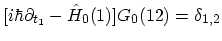, equation (3.44) and (3.45) can be rewritten as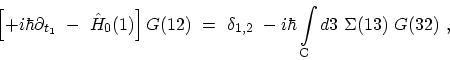(3.60)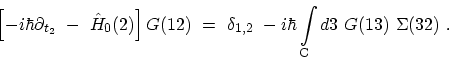(3.61)

Note that the singular part of the self-energy on the contour, which corresponds to the HARTREE self-energy (Section 3.6), does not appear explicitly in the kinetic equations, but is included in the potential energy of the single-particle HAMILTONian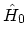, see (F.7).

Using the LANGRETH rules (Table 3.1) and fixing the time arguments of the GREEN's functions in (3.60) and (3.61) at opposite sides of the contour, one obtains the KADANOFF-BAYM equations [93,203]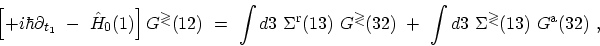(3.62)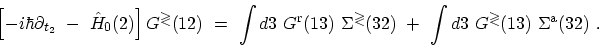(3.63)

One should note that the delta-function term in (3.60) and (3.61) vanishes identically, because the time-labels required in the construction of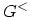and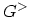are, by the definition on different branches of the contour.

The KADANOFF-BAYM equations determine the time evolution of the GREEN's functions, but they do not determine the consistent initial values. This information is contained in the original DYSON equations (3.44) and (3.45), and lost in the derivation. To have a closed set of equations, the KADANOFF-BAYM equations must be supplemented with DYSON equations for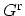and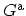. By subtracting (3.63) from (3.62), one finds the equation satisfied by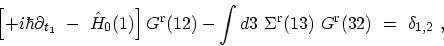(3.64)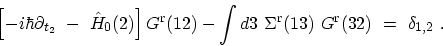(3.65)

Similar relations hold for the advanced GREEN's functions. M. Pourfath: Numerical Study of Quantum Transport in Carbon Nanotube-Based Transistors